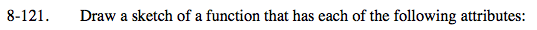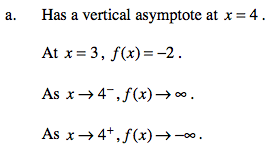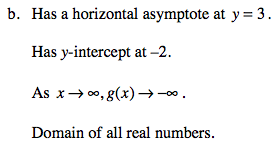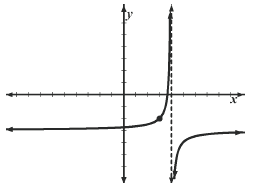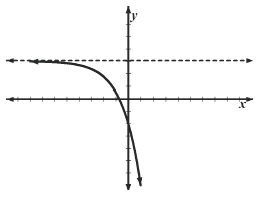### Home > PC > Chapter 8 > Lesson 8.2.4 > Problem8-121

8-121.
1.Draw a sketch of a function that has each of the following attributes: Homework Help ✎

1. Has a vertical asymptote at x = 4.

At x = 3, f(x) = −2.

As x → 4, f(x) → ∞.

As x → 4+, f(x) → −∞

2. Has a horizontal asymptote at y = 3.

Has y-intercept at −2.

As x → ∞, g(x) → −∞.

Domain of all real numbers.